# Trapping Rain Water

Key takeaway: A popular interview problem to learn optimization using various approaches. All the solutions are worth exploring, where the two-pointers idea helps to reach the efficient solution in place.

### Let’s understand the problem

Given n non-negative integers representing an elevation map where the width of each tower is 1, write a program to compute how much water it can trap after raining.

Example 1

Input: height[] = [2, 0, 1, 0, 2], Output: 5

Explanation: Trapped water = 2 x 1 + 1 x 1 + 2 x 1 = 5 (Area of the blue region in the following diagram)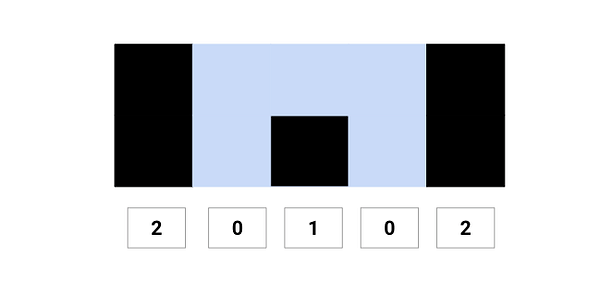Example 2

Input: height[] = [0, 1, 0, 2, 1, 0, 1, 3, 2, 1, 2, 1], Output: 6

Explanation: Trapped water = 1 x 1 + 1 x 1 + 2 x 1 + 1 x 1 + 1 x 1 = 6 (Area of the blue region in the following diagram)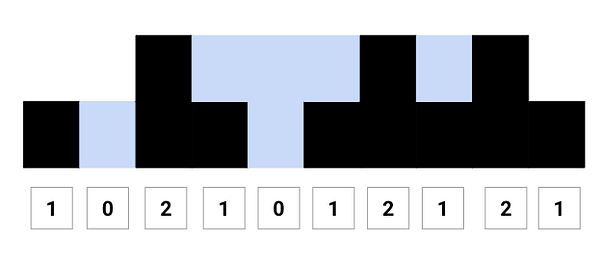### Discussed solution approaches

• A brute force solution using nested loops
• Solution using extra memory (Dynamic Programming)
• Solution using the stack data structure
• Efficient solution using two pointers approach

### A brute force solution  using nested loops

Solution Idea

One basic idea would be to scan the height[] array and sum the water trapped at each tower. The height of water trapped at any tower height[i] = minimum of maximum height of towers on both the sides minus its height, i.e., min (max tower height in the left, max tower height in the right) - height[i]

Solution Steps

• We declare and initialize the variable trappedWater to store the total trapped water.
• Now scan the height[] from from i = 0 to n-1. Inside the loop, we declare and initialize the variables leftMaxHeight and rightMaxHeight to store the maximum height of the tower on both sides of the current tower.
• For each tower height[i], we calculate the maximum height of the tower from current index i up to the left end. We store this value in the variable leftMaxHeight.

``````for(int k = i; k >= 0; k = k - 1)
leftMaxHeight = max(height[k], leftMaxHeight)
``````
• For each tower height[i], we calculate the maximum height of the tower from current index i up to the right end. We store this value in the variable rightMaxHeight.

``````for(int j = i; j < n; j = j + 1)
rightMaxHeight = max(height[j], rightMaxHeight)
``````
• Now we update the total amount of water by using the above formula.

``````trappedWater = trappedWater + min(leftMaxHeight, rightMaxHeight) - height[i]
``````
• By end of the above loop, we return the value of the variable trappedWater.

Solution Pseudocode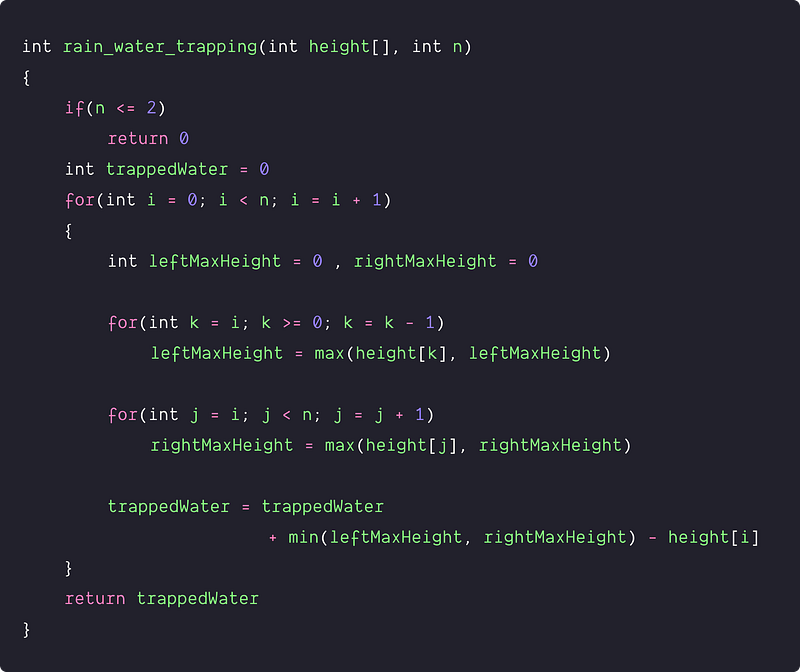Time and space complexity analysis

We are using nested loops where the outer loop is scanning the height[] array, and two inner loops are finding rightMaxHeight and leftMaxHeight. So every iteration of the outer loop, we are traversing each element via inner loops. Time Complexity = O(n * n) = O(n^2), Space Complexity = O(1)

### Solution using extra memory (Dynamic Programming)

Solution Idea

Can we improve the efficiency further and solve the problem in O(n)? Is it possible to remove the separate inner loop for finding the leftMaxHeight and rightMaxHeight? Let's think!

If the values of leftMaxHeight and rightMaxHeight are known for every tower then we can solve this problem in a single scan of the height[i] array. But how can we do this? One idea would be to pre-calculate leftMaxHeight and rightMaxHeight for each tower height[i] and store them in separate extra memory.

Suppose we take left[n] and right[n] to store the values where: left[i] = leftMaxHeight of the tower height[i], right[i] = rightMaxHeight of the tower height[i]

Can we do the pre-processing and store values in linear time? Here is an idea:

• Suppose we know the value of left[i-1], then we can easily calculate the left[i] in O(1). Here is the formula => left[i] = max (left[i-1], height[i]) (Think)
• Similarly, if we know the value of right[i+1], then we can easily calculate the right[i] in O(1). Here is the formula => right[i] = max (right[i+1], height[i]) (Think)

This is a dynamic programming approach where we are using the stored solution of the smaller problem to get the solution of the larger problem.

Solution Steps

• We create two arrays left[n] and right[n]
• Now run a loop from left to right and fill the left[n] array. At every iteration i, we store the maximum element that occurred up to that point in left[i]. (Think!)

``````left = height
for(int i = 1; i < n; i = i + 1)
left[i] = max(left[i-1], height[i])
``````
• Similarly, run a loop from right to left, and fill the right[n] array. At every iteration, store the maximum element that occurred up to that point in the right[i]. (Think!)

``````right[n-1] = height[n-1]
for(int i = n-2; i >= 0; i = i - 1)
right[i] = max(right[i+1], height[i])
``````
• Now, traverse the height[] array and calculate the total amount of water.

``````int trappeWater = 0
for(int i = 0; i < n; i = i + 1)
trappedWater = trappedWater + min(left[i], right[i]) - height[i]
``````
• Return the value stored in the variable trappedWater.

Solution Pseudocode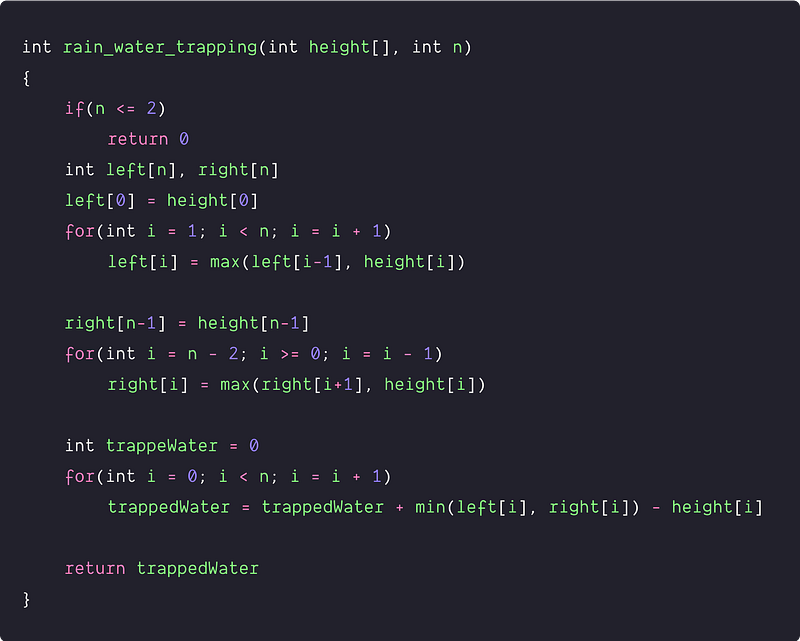Time and space complexity analysis

In the above pseudocode, we are running three independent loops of size n. To find rightMaxHeight and leftMaxHeight, we used two separate loops. And, to traverse the array, also we used one loop. So, time complexity = O(n) + O(n) = O(n).

We used two extra arrays of size n. So space complexity = O(n) + O(n) = O(n)

### Solution using the stack data structure

Solution Idea

Can we solve this problem in a single scan? Let's explore.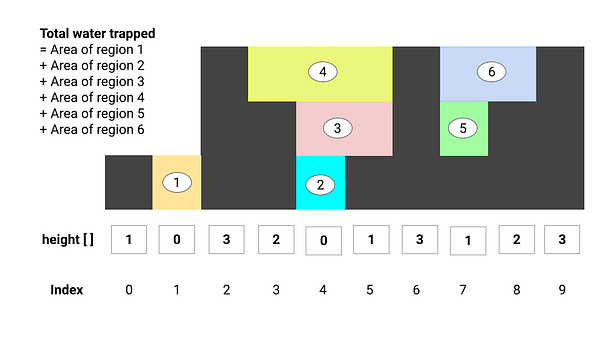• Area of region 1 = area confined between index 2 and 0
• Area of region 2 = area confined between index 5 and 3
• Area of region 3 = area confined between index 6 and 3
• Area of region 4 = area confined between index 6 and 2
• Area of region 5 = area confined between index 8 and 6
• Area of region 5 = area confined between index 9 and 6

Did we observe some patterns? The idea would be to track the area confined between the current tower and all the previous smaller towers in the sequence. We can use a stack to track the indices of the previous smaller towers. (Think!)

Solution Steps

• We declare a stack S to store the indices of the towers.
• Now we scan the height[n] using a loop variable curr < n
• If we found a current tower larger than that tower at the top of the stack, we can say that the tower at the top of the stack is confined between the current tower and a previous tower in the stack. Hence, we pop the stack and add water trapped between towers to the total water trapped. We can continue this step in a loop until we find the current tower smaller than the tower at the top of the stack.

Water trapped between towers = Area of the rectangular region formed by the current tower, popped tower, and tower at the top of the stack

• Region length = (current index - index of the top stack element - 1)
• Region height = min (height of the current tower, height of tower at top of the stack) - the height of the popped tower
• Water trapped = Region length x Region height
• If the current tower is smaller than or equal to the tower at the top of the stack, we push the index of the current tower to the stack and move to the next tower. It means the current tower is confined with the tower at the top of the stack.

Solution Pseudocode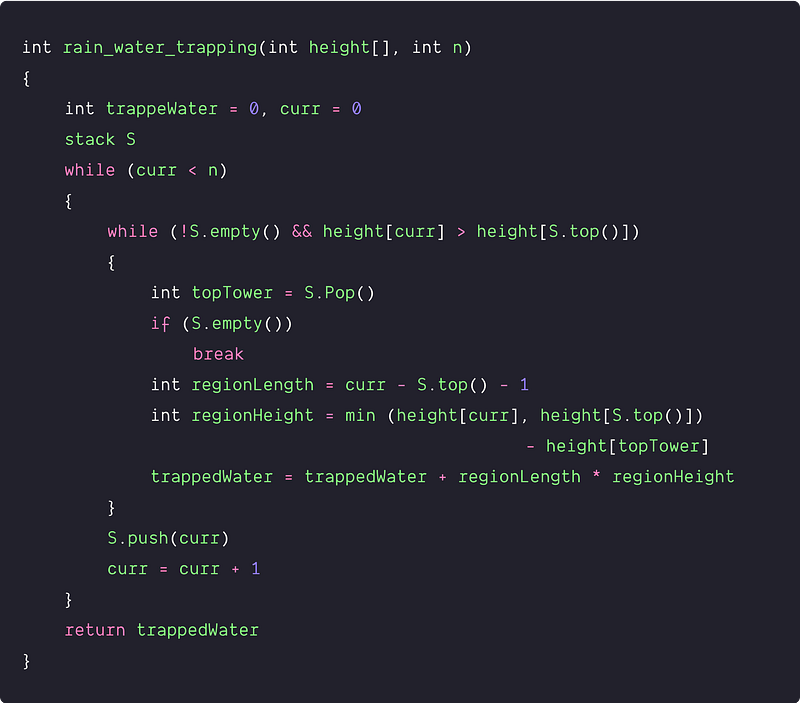Time and space complexity analysis

We are doing a single traversal of the height[] array. In the worst case, we are processing each tower twice using a stack i.e. one push() and one pop() operation. (Think!) Both push() and pop() operations take O(1) time in the worst case. So, time complexity = O(n).

In the worst-case, the stack can take up to n elements. Space complexity = O(n)

Note: If(height[curr] > height[S.top()]), then the tower at the top of the stack is smaller than the previous tower in the stack and current tower. In such a scenario, it would form a colored rectangular region and trapped water is equal to the area of that region.

### An efficient solution using two pointers approach

Solution Idea

In the last two approaches, we are using extra spaces to get the solution. Can we solve this problem in a single scan without using extra space? Let's explore!

Based on the formula used in the 2nd approach, the water trapped by any tower depends on the minimum of maximum height of towers on both sides. So instead of maintaining two arrays of size n for storing leftMaxHeight and a rightMaxHeight, can we think to maintain two variables to store the maximum till a given point? Think!

Suppose we search from both the left and right end by maintaining two pointers left and right separately. If there is a larger tower at the right end, then the water trapped would be dependant on the tower's height in the direction from left to right. Similarly, if the tower at the right end is smaller, then the water trapped would be dependant on the tower's height in the direction from right to left. (Think!)

We first calculate the water trapped on smaller elements out of height[left] and height[right], then move the pointer associated with the smaller element. We move the pointers till the left doesn't cross right. In terms of analogy, we can think of height[left] and height[right] as a wall of a partial container where we fix the higher one and flow water from the lower part.

Solution Steps

• We declare and Initialise left and right pointers.
• Also declare and Initialise variables trappedWater, leftMaxHeight, and rightMaxHeight.

``````int trappedWater = 0
int leftMaxHeight = 0, rightMaxHeight = 0
int left = 0, right = n - 1
``````
• Now run a loop to scan the array i.e. while (left <= right)

1. If (height[left] < height[right]) and (height[left] < leftMaxHeight), then we calculate the trapped water stored by the both towers and increase the left pointer. But if (height[left] > leftMaxHeight), then we update value of leftMaxHeight and increase the left pointer.
``````if (height[left] < height[right])
{
if (height[left] > leftMaxHeight)
leftMaxHeight = height[left]
else
trappedWater = trappedWater + leftMaxHeight - height[left]
left = left + 1
}
``````
1. If (height[left] > height[right]) and height[right] < rightMaxHeight) then we calculate the trapped water stored by the both towers and decrease the right pointer. But if (height[right] > rightMaxHeight), then we update value of rightMaxHeight and decrease the right pointer.
``````if (height[left] > height[right])
{
if (height[right] > rightMaxHeight)
rightMaxHeight = height[right]
else
trappedWater = trappedWater + rightMaxHeight - height[right]
right = right - 1
}
``````
• When left > right, then we exit the loop and return the value stored in the trappedWater.

Solution Pseudocode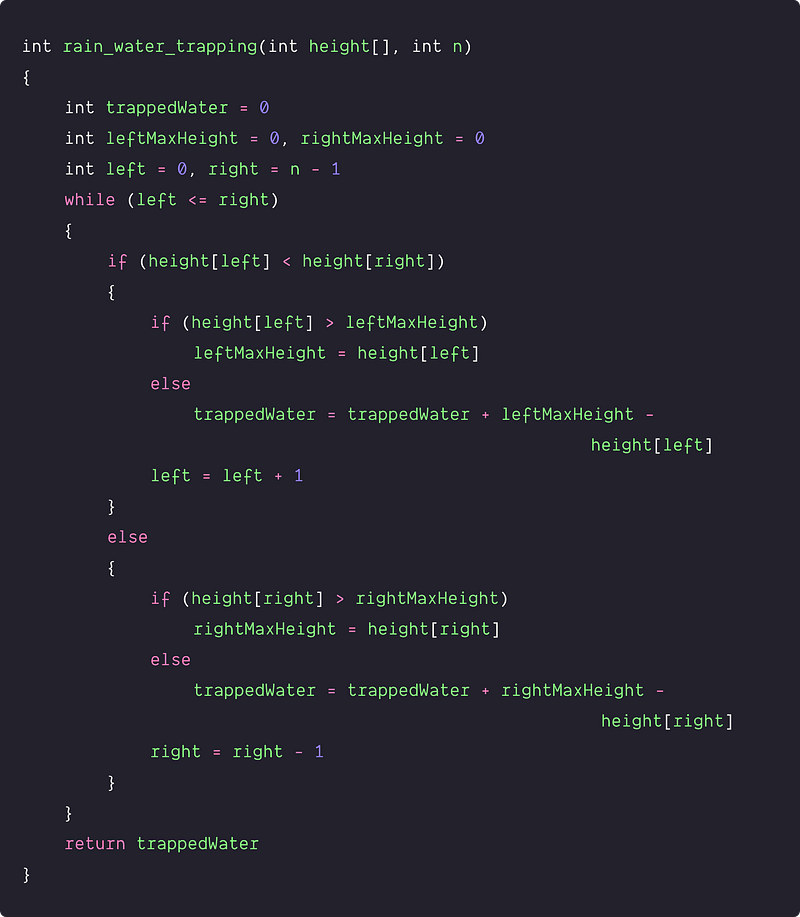Time and space complexity analysis

We are doing a single scan to traverse the array from both ends. After each comparison, we are moving either the left pointer or the right pointer. So in the worst case, we need to do O(n) operations.

Time complexity = O(n), Space complexity = O(1), only constant space required for variables and pointers.

### Critical ideas to think!

• Can we find some other approach to solve this problem in O(n) time and O(1) space? Here is a hint: We first search the maximal tower in height [] and split the array into two halves around it. Now we do two traversals: one from the leftmost tower to the highest tower and another one from the rightmost to the highest tower.
• In the dynamic programming approach, can we save one pass? Here is a hint: Use one array to keep track of maximum height on one side, i.e., left or right. On the other side, we can use a variable to keep track of maximum height so far and process it on the fly during the process when we calculate the volume of water in each tower.
• In the stack-based approach, what would be the best and worst-case scenario?
• In the 4th approach, can we compare leftMaxHeight and rightMaxHeight to decide which pointer to move?
• Let's try a different version of the problem: Given an m x n integer matrix height[][] representing the height of each unit cell in a 2D elevation map, return the volume of water it can trap after raining.

### Comparisons of time and space complexities

• Brute force approach: Time = O(n²), Space = O(1)
• Using Dynamic Programming: Time = O(n), Space = O(n)
• Using Stack: Time = O(n), Space = O(n)
• Using two pointers: Time = O(n), Space = O(1)

### Similar coding questions to practice

Thanks to Navtosh Kumar for his contribution in creating the first version of the content. Please write comments if you find an error or you want to share more insights about the topic. Enjoy learning, Enjoy coding, Enjoy algorithms!

Get well-designed application and interview centirc content on ds-algorithms, machine learning, system design and oops. Content will be delivered weekly.